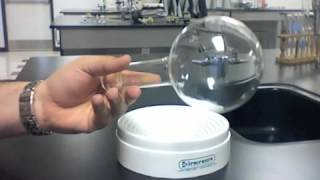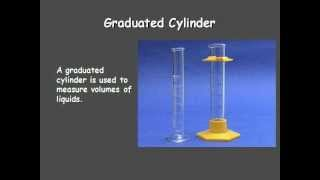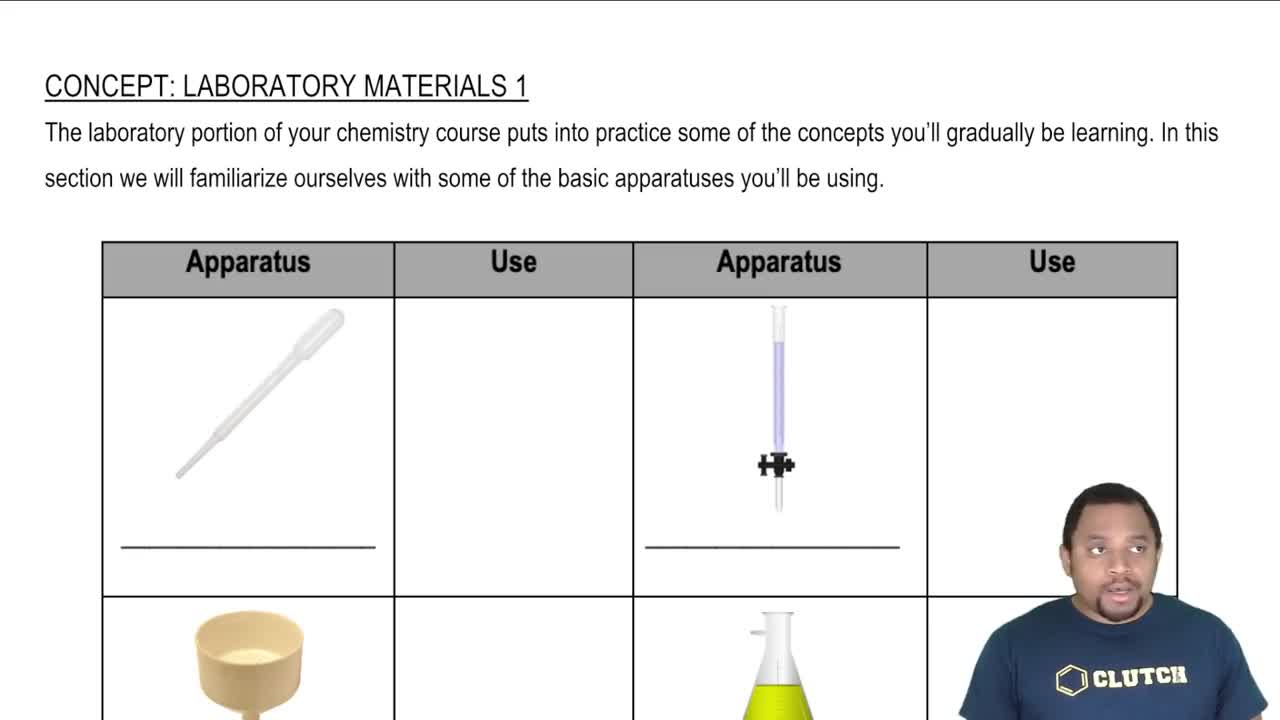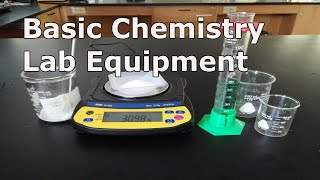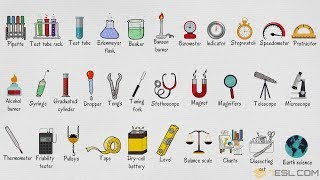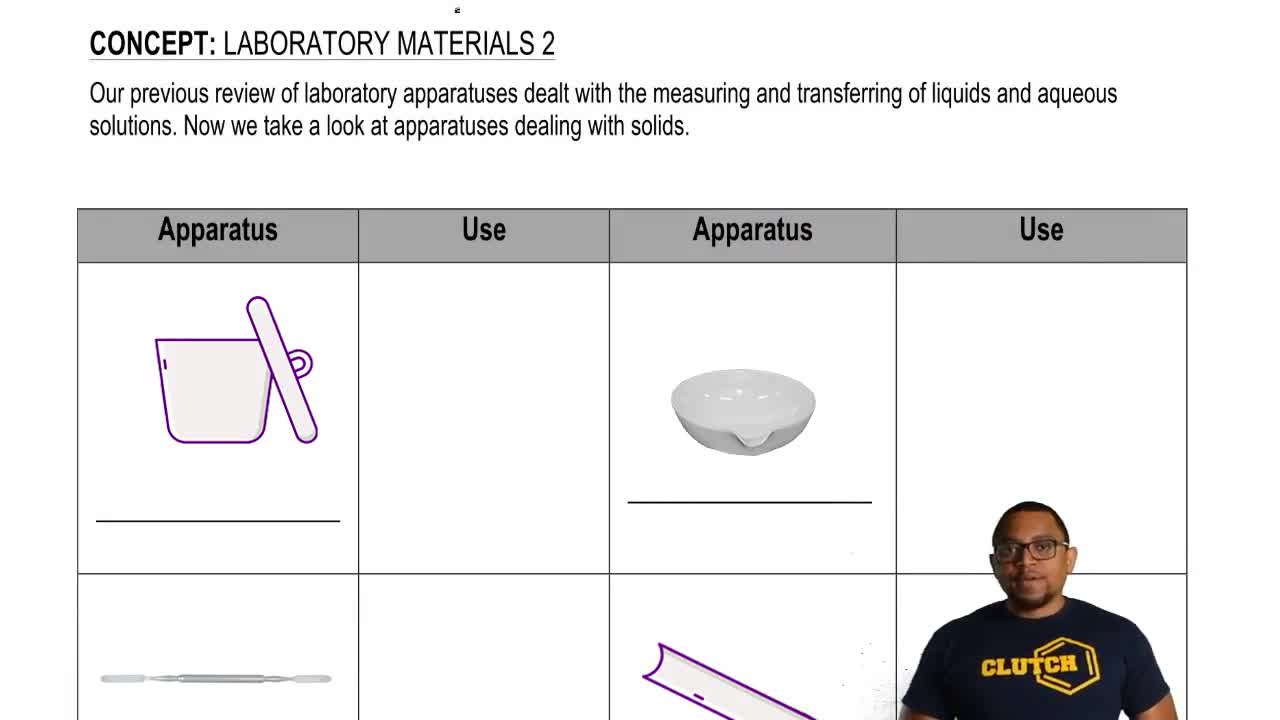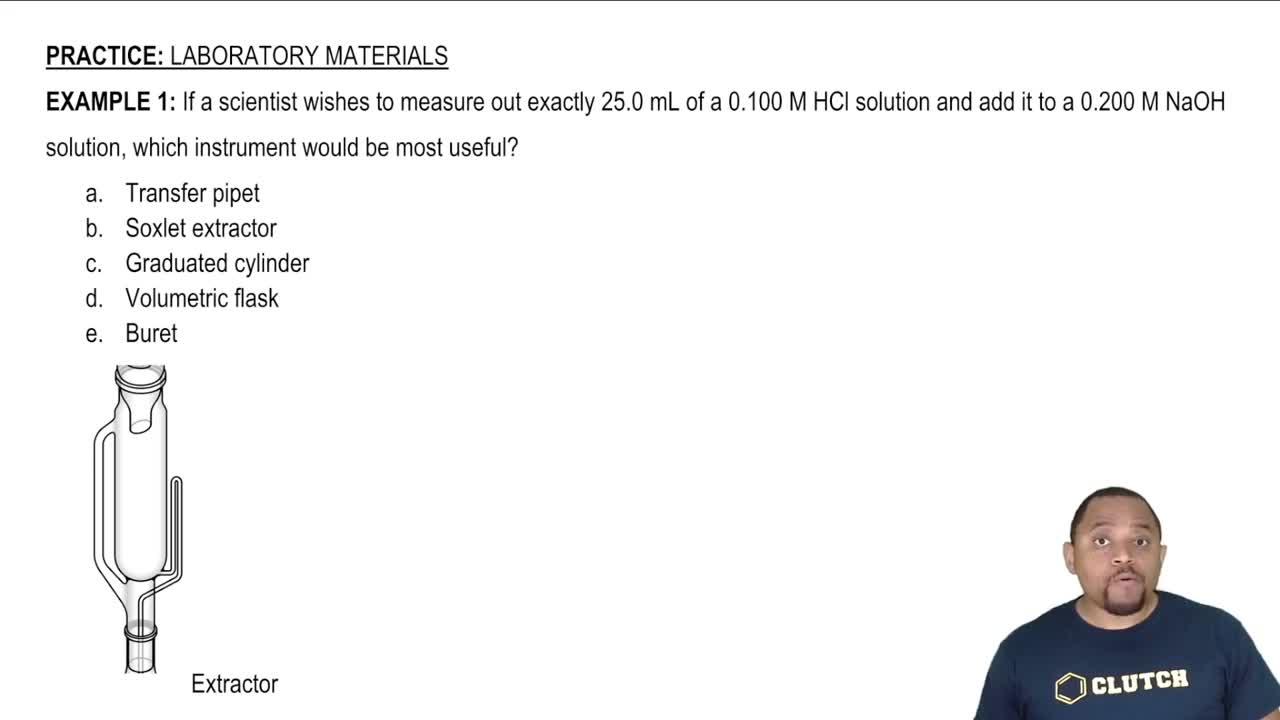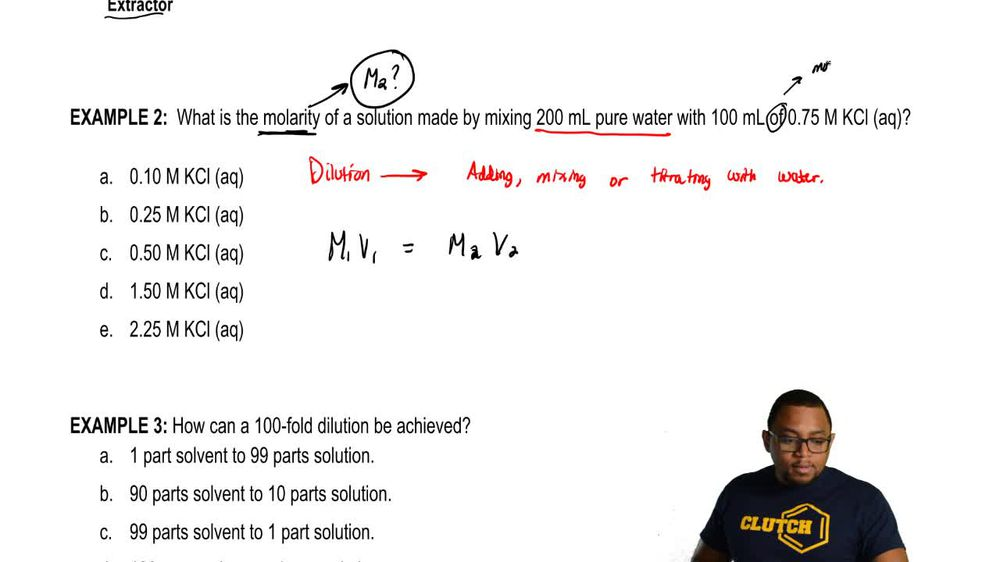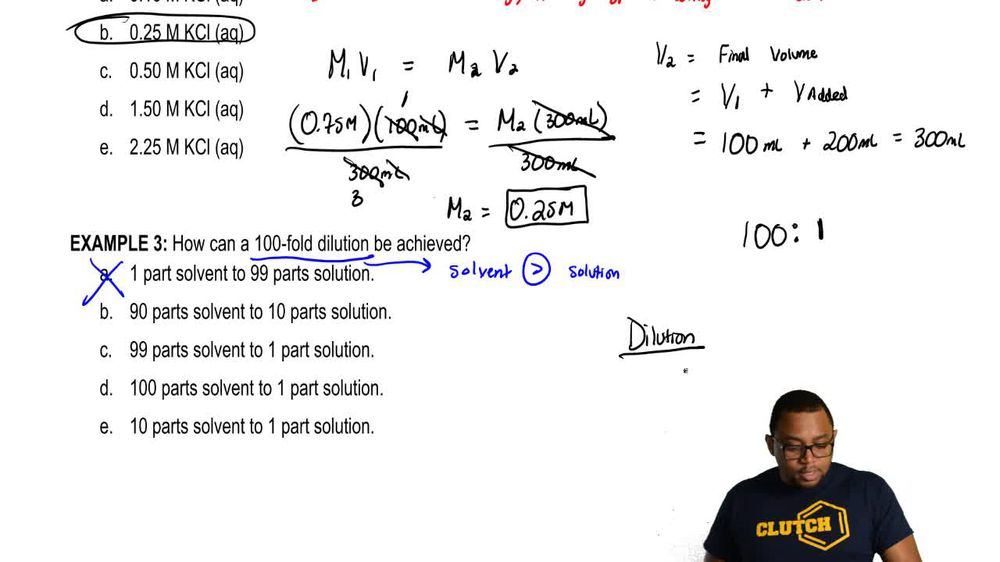Start typing, then use the up and down arrows to select an option from the list.
1. 4. BONUS: Lab Techniques and Procedures2. Laboratory Materials# Laboratory Materials

by Jules Bruno
45 views
0
So for a final example question on this page, we're gonna deal with a dilution. Now, it's one thing to just use the dilution equation to solve within the classroom. But it's another thing when you're in the lab and asked to do a dilution yourself, the illusions could be a bit tricky. So the approach we wanna take is this. So we want to create 100 fold dilution. Okay, so just remember, in a dilution that means that are solvent will have to be larger than our solution. Okay, so we have to put that out there first. So knowing this, we know that they won't work because in a the solvent is less than our solution. So that's not gonna work. And when it comes to a dilution where they want us to do 100 fold dilution, so that means it's gonna be a ratio of 100 to 1. Well, what exactly is the 100 referring to? And what exactly is the one referring to when it comes to a dilution we're gonna say Here it is the solvent plus solution and it's ratio to the amount of solution. Okay, so that's what the 100 toe one is referring to 100 fold. Dilution is 100 to 1. Maybe we have ah 100 when it comes to adding up the amount of solvent and solution together compared to one for the solution. So here we know that a is out. So if we take a look at the other options, what do we have for B? We have 90 and 10 So this would be plus 10 and then this would be 10th. So that would total up to 100 to 10. And this would represent a If you divide both by 10 this would represent a 10 to dilution or a tenfold dilution, which is not what we want next for See, we have 99 parts solvent plus one part solution solution. So that would be 99 plus one and then one. So that would be 100 to 1. This would represent our 100 fold dilution If we take a look at the last ones here we have 100 plus one toe, one that be 101 toe one. Not quite what we want. This would be 101 full dilution which is not what we want. And then the last one would be 10 plus one toe one, so that would be 11 to 1. So this would be an 11 fold dilution. So here, just remember a dilution. When it comes to the ratio, it's the amount of solvent plus solution in ratio to the amount of solution. Hundredfold would mean that we have 100 when it comes to the amount of solvent and solution together compared to the just the amount of the solution.